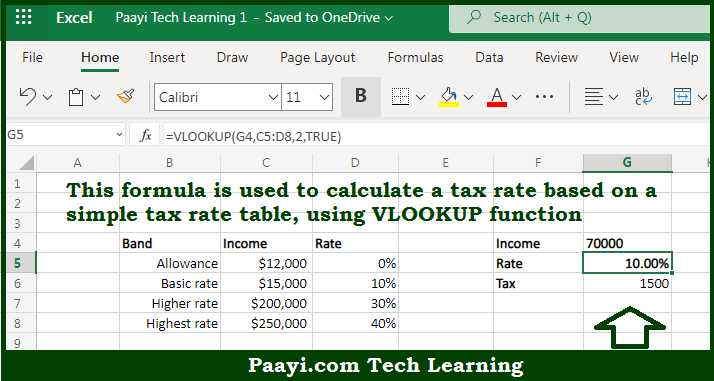# Learn How to Get Basic Tax Rate Calculation With VLOOKUP in Microsoft Excel

Written by | 0 Comments | 344 Views

In this article, you will learn how to evaluate things based on the LOOKUP function in Microsoft Excel using a single/combination(s) of functions. You will also know to Get Basic Tax Rate Calculation With VLOOKUP and see the generic formula.

Learn How to Get Basic Tax Rate Calculation With VLOOKUP in Microsoft Excel

The main purpose of this formula is to calculate a tax rate based on a simple tax rate table. Here we will learn how to get the basic tax rate calculation with the VLOOKUP function in the given data range in the workbook in Microsoft Excel. That implies, with the help of a formula based on the VLOOKUP function you can able to calculate a tax rate based on a simple tax rate table. So, with the help of this formula, you can able to get the basic tax rate calculation with the VLOOKUP function in the given data range in the workbook in Microsoft Excel.

General Formula to Get Basic Tax Rate Calculation With VLOOKUP

=VLOOKUP(amount,tax_table,2,TRUE)

The Explanation to Get Basic Tax Rate Calculation With VLOOKUPSo we know that with the help of the given formula above you can able to calculate a tax rate based on a simple tax rate table. Here we will learn how to get the basic tax rate calculation with the VLOOKUP function in the given data range in the workbook in Microsoft Excel. As we know that the VLOOKUP function needs lookup values to be in the first column of the lookup table. As the first column in the example is actually "Band", we are purposely excluding this column from the table range provided to the VLOOKUP function. So now you have learned how to get the basic tax rate calculation with VLOOKUP in the given data range in the workbook in Microsoft Excel.